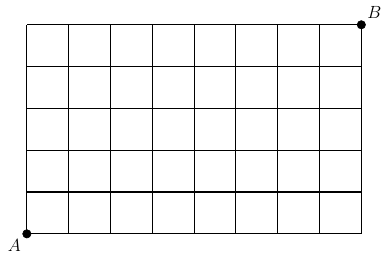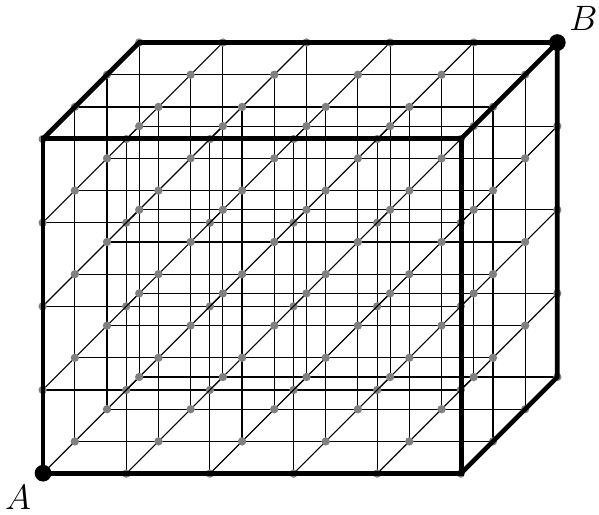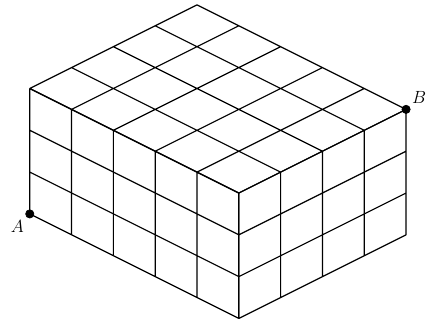###### back to index | new

Melinda has three empty boxes and $12$ textbooks, three of which are mathematics textbooks. One box will hold any three of her textbooks, one will hold any four of her textbooks, and one will hold any five of her textbooks. If Melinda packs her textbooks into these boxes in random order, find the probability that all three mathematics textbooks end up in the same box.

• In a group of nine people each person shakes hands with exactly two of the other people from the group. Let $N$ be the number of ways this handshaking can occur. Consider two handshaking arrangements different if and only if at least two people who shake hands under one arrangement do not shake hands under the other arrangement. Find $N$.

Consider an orange and black coloring of a $20\times 14$ square grid. Let $n$ be the number of coloring such that every row and column has an even number of orange square. Evaluate $\log_2 n$.

A word is an ordered, non-empty sequence of letters, such as word or wrod. How many distinct words can be made from a subset of the letters $\textit{c, o, m, b, o}$, where each letter in the list is used no more than the number of times it appears?

In the following $(8\times 5)$ grid, how many shortest routes are there from point $A$ to point $B$?In the following $5\times 4\times 3$ grid system, how many shortest routes are there from point $A$ to point $B$?In the following $5\times 4\times 3$ grid, how many shortest routes are there from point $A$ to point $B$ on its surface?There are $5$ red balls and $4$ green balls in a bag. One ball is retrieved a time until all the balls are taken out. How many possible ways are there such that all the red balls are taken out before all the green balls are taken out?

There are $5$ red balls, $4$ green balls and $3$ yellow balls in a bag. One ball is retrieved a time until all the balls are taken out. What is the probability that all the red balls are retrieved before all the green or yellow balls are retrieved?

Show that for any positive integer $n$, the value of $\frac{(n^2)!}{(n!)^{n+1}}$ is an integer.

How many distinct permutations of the letters of the word REDDER are there that do not contain a palindromic substring of length at least two? (A substring is a contiguous block of letters that is part of the string. A string is palindromic if it is the same when read backwards.)